# Field Oriented Control

AC Induction motors offer enviable operational characteristics such as robustness, reliability and ease of control. They are extensively used in various applications ranging from industrial motion control systems to home appliances. However, the use of induction motors at its highest efficiency is a challenging task because of their complex mathematical model and non-linear characteristic during saturation. These factors make the control of induction motor difficult and call for the use of a high performance control algorithms such as vector control.

## Introduction of Field Oriented Control

Scalar control such as the “V/Hz” strategy has its limitations in terms of performance. The scalar control method for induction motors generates oscillations on the produced torque. Hence to achieve better dynamic performance, a more superior control scheme is needed for Induction Motor. With the mathematical processing capabilities offered by the micro-controllers, digital signal processors and FGPA, advanced control strategies can be implemented to decouple the torque generation and the magnetization functions in an AC induction motor. This decoupled torque and magnetization flux is commonly called rotor Flux Oriented Control (FOC).

Field Oriented Control describes the way in which the control of torque and speed are directly based on the electromagnetic state of the motor, similar to a DC motor. FOC is the first technology to control the “real” motor control variables of torque and flux. With decoupling between the stator current components (magnetizing flux and torque), the torque producing component of the stator flux can be controlled independently. Decoupled control, at low speeds, the magnetization state of motor can be maintained at the appropriate level, and the torque can be controlled to regulate the speed.
“FOC has been solely developed for high-performance motor applications which can operate smoothly over the wide speed range, can produce full torque at zero speed, and is capable of quick acceleration and deceleration.”

## Working Principle of Field Oriented Control

The field oriented control consists of controlling the stator currents represented by a vector. This control is based on projections that transform a three phase time and speed dependent system into a two coordinate (d and q frame) time invariant system. These transformations and projections lead to a structure similar to that of a DC machine control. FOC machines need two constants as input references: the torque component (aligned with the q coordinate) and the flux component (aligned with d coordinate).
The three-phase voltages, currents and fluxes of AC-motors can be analyzed in terms of complex space vectors. If we take ia, ib, ic as instantaneous currents in the stator phases, then the stator current vector is defined as follow: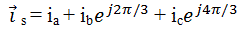Where, (a, b, c) are the axes of three phase system.

This current space vector represents the three phase sinusoidal system. It needs to be transformed into a two time invariant coordinate system. This transformation can be divided into two steps:
(a, b, c) → (α, β) (the Clarke transformation), which gives outputs of two coordinate time variant system.
(a, β) → (d, q) (the Park transformation), which gives outputs of two coordinate time invariant system.
The (a, b, c) → (α, β) Projection (Clarke transformation)
Three-phase quantities either voltages or currents, varying in time along the axes a, b, and c can be mathematically transformed into two-phase voltages or currents, varying in time along the axes α and β by the following transformation matrix:Assuming that the axis a and the axis α are along same direction and β is orthogonal to them, we have the following vector diagram: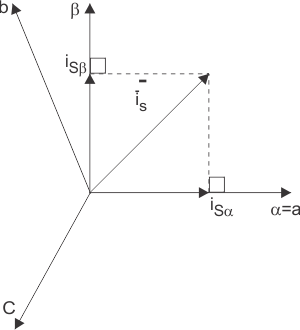The above projection modifies the three phase system into the (α, β) two dimension orthogonal system as stated below:But these two phase (α, β) currents still depends upon time and speed.
The (α, β) → (d.q) projection (Park transformation)
This is the most important transformation in the FOC. In fact, this projection modifies the two phase fixed orthogonal system (α, β) into d, q rotating reference system. The transformation matrix is given below: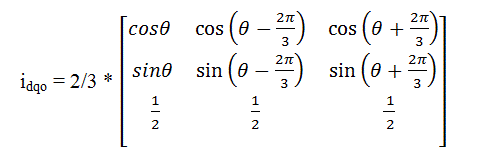Where, θ is the angle between the rotating and fixed coordinate system.
If you consider the d axis aligned with the rotor flux, Figure 2 shows the relationship from the two reference frames for the current vector:Where, θ is the rotor flux position. The torque and flux components of the current vector are determined by the following equations: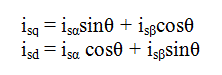These components depend on the current vector (α, β) components and on the rotor flux position. If you know the accurate rotor flux position then, by above equation, the d, q component can be easily calculated. At this instant, the torque can be controlled directly because flux component (isd) and torque component (isq) are independent now.

## Basic Module for Field Oriented Control

Stator phase currents are measured. These measured currents are fed into the Clarke transformation block. The outputs of this projection are entitled i and i. These two components of the current enter into the Park transformation block that provide the current in the d, q reference frame. The isd and isq components are contrasted to the references: isdref (the flux reference) and isqref (the torque reference). At this instant, the control structure has an advantage: it can be used to control either synchronous or induction machines by simply changing the flux reference and tracking rotor flux position. In case of PMSM the rotor flux is fixed determined by the magnets so there is no need to create one. Therefore, while controlling a PMSM, isdref should be equal to zero. As induction motors need a rotor flux creation in order to operate, the flux reference must not be equal to zero. This easily eliminates one of the major shortcomings of the “classic” control structures: the portability from asynchronous to synchronous drives. The outputs of the PI controllers are Vsdref and Vsqref. They are applied to the inverse Park transformation block. The outputs of this projection are Vsαref and Vsβref are fed to the space vector pulse width modulation (SVPWM) algorithm block. The outputs of this block provide signals that drive the inverter. Here both Park and inverse Park transformations need the rotor flux position. Hence rotor flux position is essence of FOC.
The evaluation of the rotor flux position is different if we consider the synchronous or induction motor.

1. In case of synchronous motor(s), the rotor speed is equal to the rotor flux speed. Then rotor flux position is directly determined by position sensor or by integration of rotor speed.
2. In case of asynchronous motor(s), the rotor speed is not equal to the rotor flux speed because of slip; therefore a particular method is used to evaluate rotor flux position (θ). This method utilizes current model, which needs two equations of the induction motor model in d,q rotating reference frame.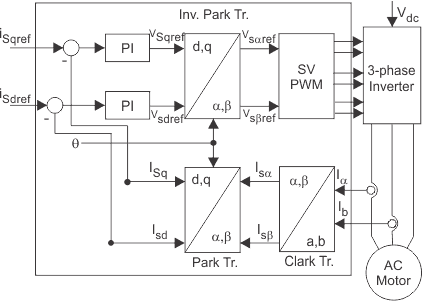Simplified Indirect FOC Block Diagram

## Classification of Field Oriented Control

FOC for the induction motor drive can be broadly classified into two types: Indirect FOC and Direct FOC schemes. In DFOC strategy rotor flux vector is either measured by means of a flux sensor mounted in the air-gap or by using the voltage equations starting from the electrical machine parameters. But in case of IFOC rotor flux vector is estimated using the field oriented control equations (current model) requiring a rotor speed measurement. Among both schemes, IFOC is more commonly used because in closed-loop mode it can easily operate throughout the speed range from zero speed to high-speed field-weakening.

## Advantages of Field Oriented Control

1. Improved torque response.
2. Torque control at low frequencies and low speed.
3. Dynamic speed accuracy.
4. Reduction in size of motor, cost and power consumption.